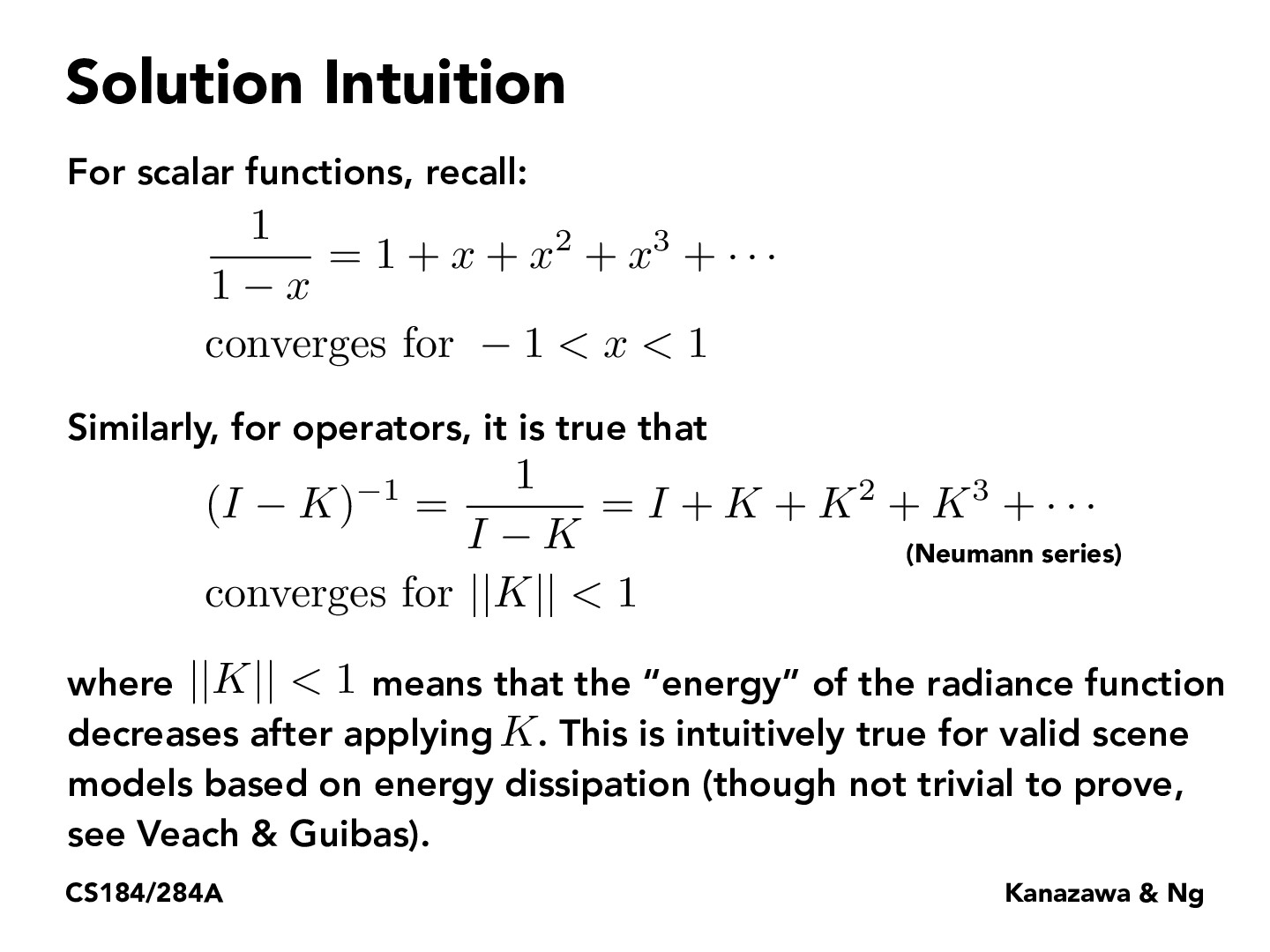Lecture 13: Global Illumination & Path Tracing (41)philippe-eecs

The first equation comes from a geometric sequence sum. https://en.wikipedia.org/wiki/Geometric_series

If you take the initial sum (1 + x ... + x^ n) = s

and take the initial sum times x (x + x^2 + ... + x^ {n + 1}) = sx

And you then subtract, you get s(1 - x) = 1 - x^{n + 1}

s = (1 - x^{n + 1})/(1 - x)

taking the limit as n approaches infinity will give you 1/(1 - x) as long as -1 < x < 1. Otherwise it goes to infinitychristineelu

The Neumann series applies for the light rendering equation because an object can only reflect at most as much light as it receives. This is because light energy can be absorbed into dark materials or get converted into heat energy (conservation of energy principles).

You must be enrolled in the course to comment